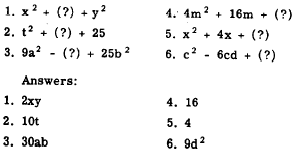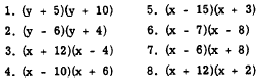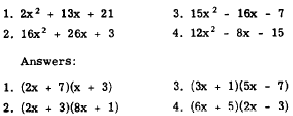Custom SearchTRINOMIAL SQUARES A trinomial that is the square of a binomial is called a TRINOMIAL SQUARE. Trinomials that are perfect squares factor into either the square of a sum or the square of a difference. Recalling that (x + y)2 = x2 + 2xy + y2 and (x - y)2 = x2 - 2xy + y2, the form of a trinomial square is apparent. The first term and the last term are perfect squares and their signs are positive. The middle term is twice the product of the square roots of these two numbers. The sign of the middle term is plus if a sum has been squared; it is minus if a difference has been squared. The polynomial 16x2 - 8xy + x2 is a trinomial in which the first term, 16x , and the last term, y2, are perfect squares with positive signs. The square roots are 4x and y. Twice the product of these square roots is 2(4x)(y) = 8xy. The middle term is preceded by a minus sign indicating that a difference has been squared. In factored form this trinomial is as follows: 16x2 - 8xy + y2 = (4x - y)2 To factor the trinomial, we simply take the square roots of the end terms and join them with a plus sign if the middle term is preceded by a plus or with a minus if the middle term is preceded by a minus. The terms of a trinomial may appear in any order. Thus, 8xy + y2 + 16x2 is a trinomial square and may be factored as follows: 8xy + y2 + 16x2 = 16x2 + 8xy + y2 = (4x + y)2 Practice problems. Among the following expressions, factor those which are trinomial squares:SUPPLYING THE MISSING TERM Skill in recognizing trinomial squares may be improved by practicing the solution of problems which require supplying a missing term. For example, the expression y2 + (?) + 16 can be made to form a perfect trinomial square by supplying the correct term to fill the parentheses. The middle term must be twice the product of the square roots of the two perfect square terms; that is, (2)(4)(y), or 8y. Check: y2 + 8y + 16 = (y + 4)2. The missing term is 8y. Suppose that we wish to supply the missing term in 16x2 + 24xy + (?) so that the three terms will form a perfect trinomial square. The square root of the first term is 4x. One-half the middle term is 12xy. Divide 12xy by 4x. The result is 3y which is the square root of the last term. Thus, our missing term is 9y2. Checking, we find that (4x + 3y)2 = 16x2 + 24xy + 9y2. Practice problems. In each of the following problems, supply the missing term to form a perfect trinomial square:OTHER TRINOMIALS It is sometimes possible to factor trinomials that are not perfect squares. Following are some examples of such trinomials, and the expressions of which they are products:It is apparent that trinomials like these may be factored into binomials as shown. Notice how the trinomial in each of the preceding examples is formed. The first term is the square of the common term of the binomial factors. The second term is the algebraic sum of their unlike terms times their common term. The third term is the product of their unlike terms, Such trinomials may be factored as the product of two binomials if there are two numbers such that their algebraic sum is the coefficient of the middle term and their product is the last term. For example, let us factor the expression x2 - 12x + 32. If the expression is factorable, there will be a common term, x, in each of the binomial factors. We begin factoring by placing this term within each set of parentheses, as follows:             (x  )(x  ) Next, we must find the other terms that are to go in the parentheses. They will be two numbers such that their algebraic sum is -12 and satisfy the conditions. Thus, the following expression results : X2 - 12x + 32 = (x - 8)(x - 4) It is of value in factoring to note some useful facts about trinomials. If both the second and third terms of the trinomial are positive, the signs of the terms to be found are positive as in example 1 of this section. If the second term is negative and the last is positive, both terms to be found will be negative as in example 2. If the third term of the trinomial is negative, one of the terms to be found is positive and the other is negative as in examples 3 and 4. Concerning this last case, if the second term is positive as in example 3, the positive term in the factors has the greater numerical value. If the second term is negative as in example 4, the negative term in the factors has the greater numerical value. It should be remembered that not all trinomials are factorable. For example, x2 + 4x + 2 cannot be factored since there are no two rational numbers whose product is 2 and whose sum is 4. Practice problems. Factor completely, in the following problems:Answers:Thus far we have considered only those expressions in which the coefficient of the first term is 1. When the coefficient of the first term is other than 1, the expression can be factored as shown in the following example: 6x2 - x - 2 = (2x + 1)(3x - 2) Although this result can be obtained by the trial and error method, the following procedure saves time and effort. First, find two numbers whose sum is the coefficient of the second term (-1 in this example) and whose product is equal to the product of the third term and the coefficient of the first term (in this example, (6)(-2) or -12). By inspection, the desired numbers are found to be -4 and +3. Using these two numbers as coefficients for x, we can rewrite the original expression as 6x2 - 4x + 3x - 2 and factor as follows:Practice problems. Factor completely, in the following problems: